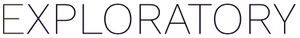# How to normalize numeric values

#1

To normalize the numeric value, you can select “normalize” from the Column Header Menu.

Work with Numeric Function -> normalize

This opens Create Calculation Dialog like below.

And this will create normalized (mean:0, SD:1)

### normalize

Syntax
normalize( `<column_num>` , center = `<logical>` , scale = `<logical>` )

Arguments

• center (Optional) - The default is TRUE. Either a logical value of TRUE or FALSE, or a numeric value. If it is TRUE then centering is done by subtracting the mean (omitting NAs) of the column values. If center is FALSE, no centering is done.
• scale (Optional) - The default is TRUE. Either a logical value of TRUE or FALSE, or a numeric value. When it is TRUE and center is TRUE, scaling is done by dividing by the standard deviation. In other words, it will end up as calculating `(x - mean(x)) / sd(x)` . When it is TRUE and center is FALSE, scaling is done by dividing by the root-mean-square, which is same as calculating `x / sqrt(sum(x^2)/(length(x)-1))` . When it is a numeric value, input values are devided by this numeric value. When it is FALSE, no scaling is done.